OTHERS 三月 28, 2021

## 浮点数的表示形式

IEEE 二进制浮点数算术标准（IEEE 754）是目前最广泛使用的浮点数算术标准，为许多 CPU 与浮点运算器所采用。

IEEE 754 标准中，定义了五个基本的浮点数表示格式，其中包括三个二进制浮点数格式（32 位单精度、64 位双精度和 128 位四倍精度格式）和两个十进制浮点数格式。

1. 符号位（sign）
2. 指数位（exponent）
3. 小数位（fraction）

binary32 单精度 24 8 2^7-1=127
binary64 双精度 53 11 2^10-1=1023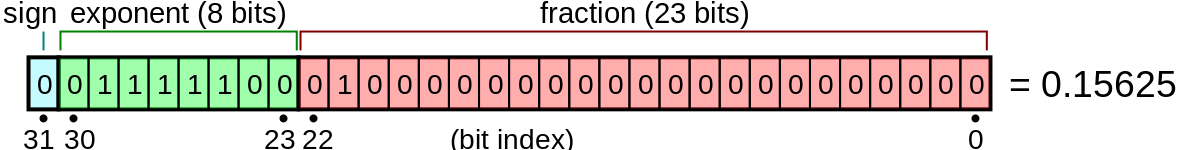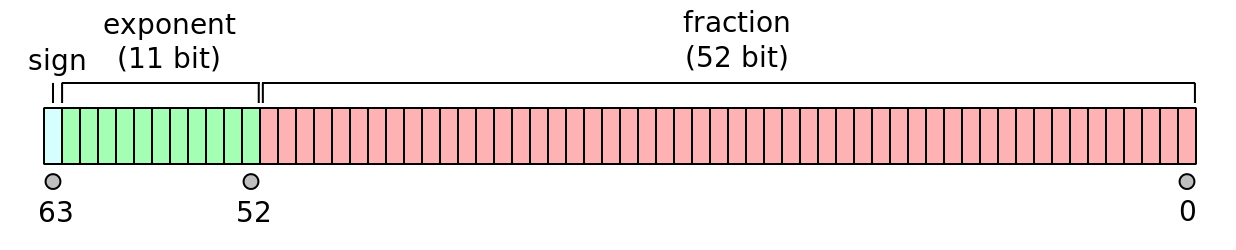## 十进制小数转换为二进制浮点数的方法

### 转换小数部分

0.125 * 2 0.25 0
0.25 * 2 0.5 0
0.5 * 2 1.0 1
0.0 * 2 0.0 0

• 2^(-1) = 1.0/2=0.5 => 1.0(2) << 2 = 0.1(2)
• 2^(-2) = 0.5/2=0.25 => 0.1(2) << 2 = 0.01(2)
• 2^(-3) = 0.25/2=0.125 => 0.01(2) << 2 = 0.001(2)
• 2^(-4) = 0.125/2=0.0625 => 0.001(2) << 2 =0.0001(2)

### 转换为指数形式

1010101.001 转换为二进制的科学计数法，为 1.010101001 * 2^6

### 填充符号位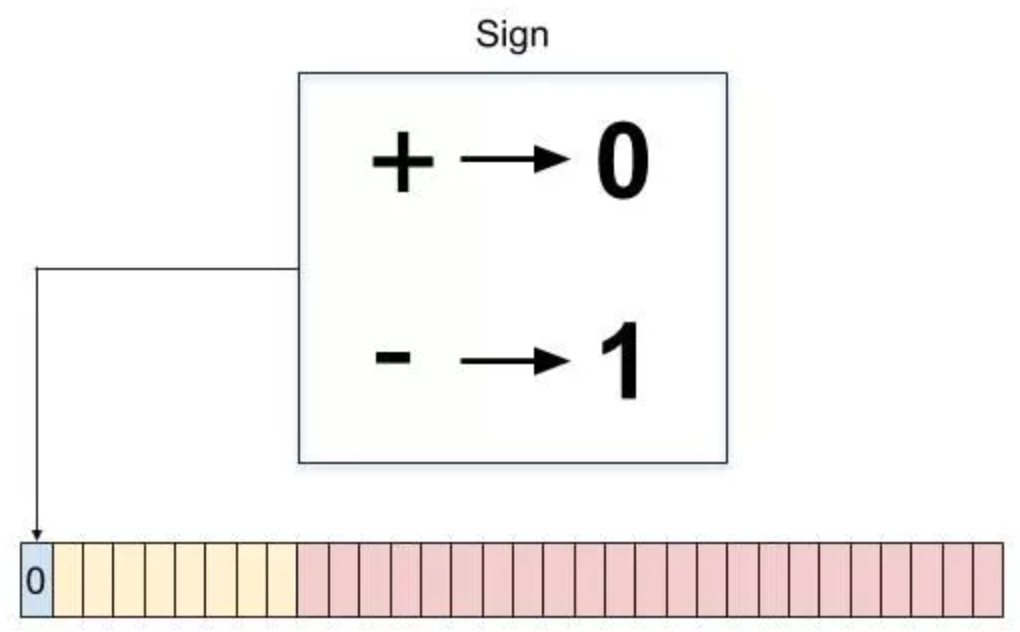### 填充指数位

1.010101001 * 2^6 的指数为 6，单精度浮点的偏移量为 127（双精度偏移量为 1023），故指数位应填入 127 + 6 = 133，转换为二进制为 10000101，直接填入指数位中：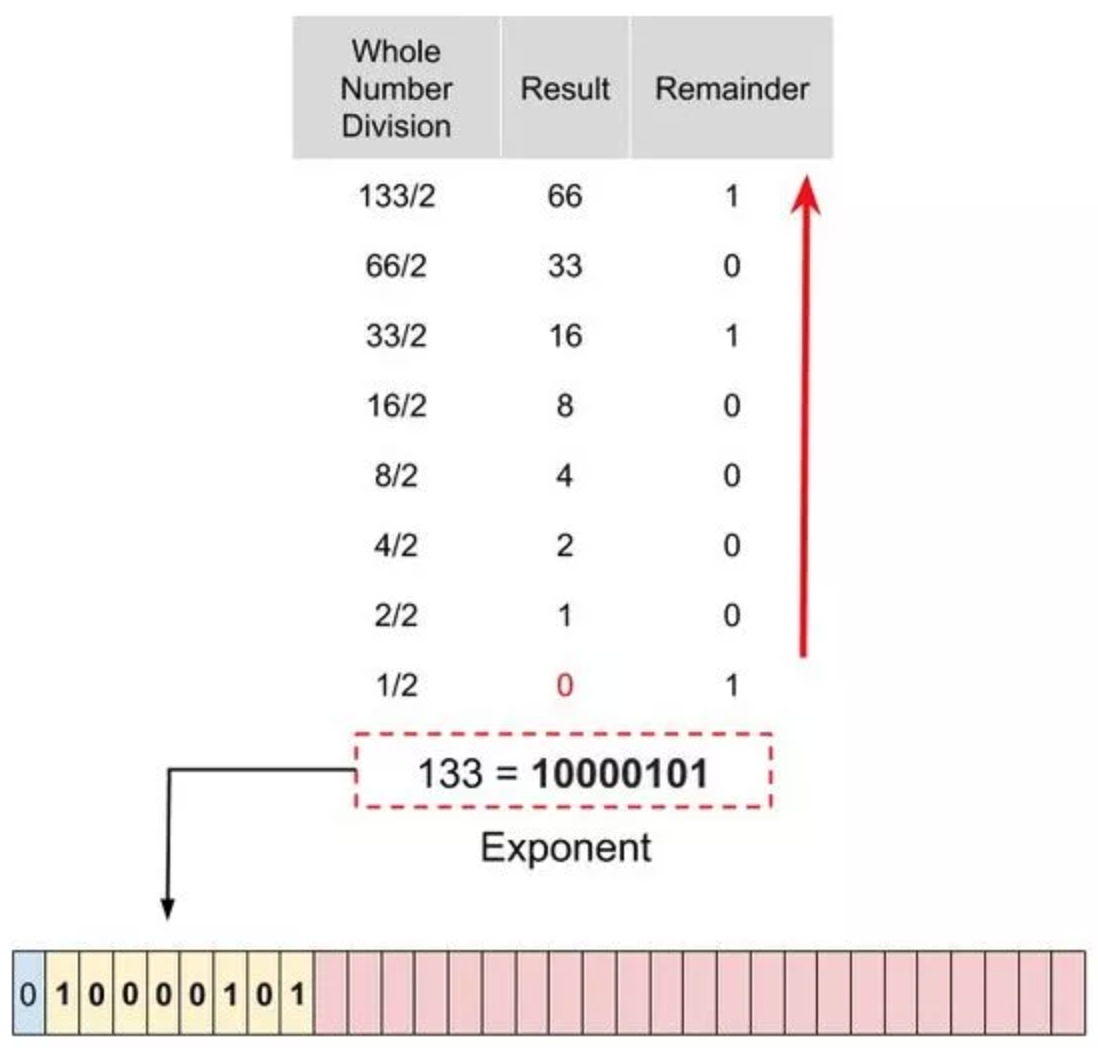### 填充小数位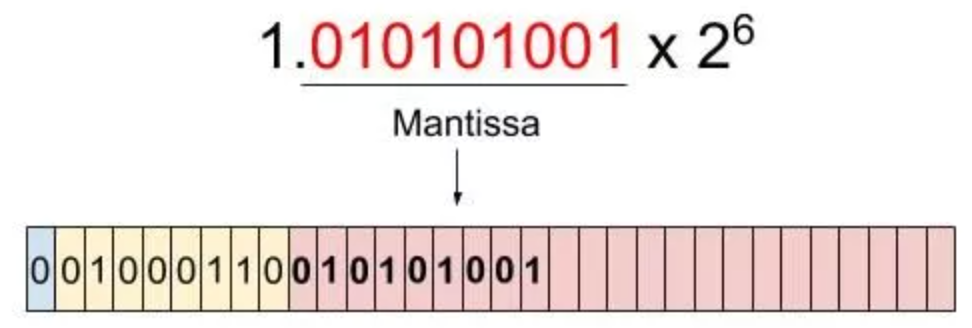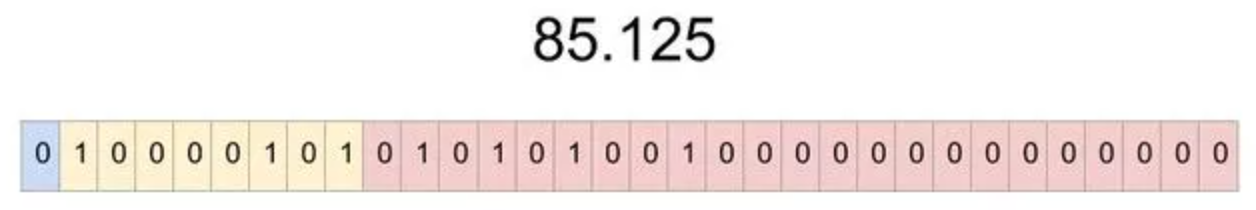## 二进制浮点数转换为十进制1.01 * 2^(-3) = 0.00101

2^(-1) 2^(-2) 2^(-3) 2^(-4) 2^(-5)
0 0 1 0 1

2^(-3) + 2^(-5) = 0.125 + 0.03125 = 0.15625

0%